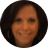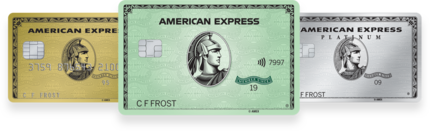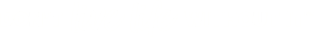United StatesChange Country

# How to Calculate Interest Rates

### Calculating interest rate can be complicated and confusing. Here are a few simple steps to calculate interest rate and credit card interest.

By Kristina Russo | American Express Credit Intel Freelance Contributor

6 Min Read | November 06, 2019 in Money## At-A-Glance

Interest rates go by different names and are calculated in different ways.

They come in two broad varieties: fixed and variable.

Calculators can help with one of the most complex forms: credit card interest.

Interest can be a funny thing. When you’re collecting it on a bank account or investment, it can make you smile. Not so much when you’re paying it to a lender. But either way, knowing how to calculate interest rates can help you know exactly how much a loan will truly cost, or how much income a particular investment will generate.

The challenge is, while simple interest rate calculations can be easy, in the real world how to calculate interest can get really complicated really fast.

#### Interest and Interest Rate Basics

Interest is the amount of money a lender charges you to borrow a set amount of money (the principal). It is the lender’s incentive to lend, and it is customarily quoted as an annual percentage of the principal. Interest can be thought of as the lender’s rate of return—so the more risk the lender expects from a borrower, the higher its incentive typically needs to be. What you, as a borrower, usually must repay is the principal amount plus calculated interest charges.

Interest rates go by many names, including borrowing rate, lending rate, mortgage rate, and lease rate. But whatever the name, interest accumulates based on the stated interest rate of a loan or on the annual percentage rate (APR) of a credit card. By law, the interest rate must be disclosed to consumers when the lending relationship begins.1

#### Where Calculating Interest Rates Starts Getting Complex

While interest rates usually are stated on an annual basis (called the nominal interest rate), they are often calculated at different frequencies depending on the terms of the loan. The different frequencies of Interest accrual tend to make real-world interest rate calculations more complicated. Some common frequencies:

• Annually
• Monthly (e.g., mortgages)
• Weekly
• Daily (e.g., credit cards)

For example, a 12 percent nominal interest rate translates to a 1 percent monthly periodic interest rate or a 0.033 percent daily periodic rate (DPR). That DPR is the 12 percent nominal rate divided by either 360 days (called “ordinary interest”) or 365 days (called “exact interest”), again, depending on the borrowing terms.

#### So, How Do You Calculate Simple Interest?

There are two main methods of calculating interest charges—simple and compound—and they can result in significant differences in interest costs. The simple interest formula, which usually results in lower overall interest costs, looks like this:

Simple Interest (SI) = Principal (P) x Nominal Rate (R) x Loan Period in Years (T)

The simple interest method multiplies the principal times the nominal interest rate times the number of years the loan will be outstanding.2

What loans typically use the simple interest method?

• Personal loans
• Auto loans
• Some student loans

#### Calculating Compound Interest

In the compound interest method, interest accrues on the outstanding principal plus any interest that was not paid during the previous compounding period—in other words, you’re charged interest on interest. The more frequent the compounding periods (represented by “N” in the formula below), the higher the resulting interest charges. Compounding is the most frequent method by which interest is calculated for mortgages, credit cards, and small business loans. The compound interest formula looks like this:5

Compound Interest (CI) = P (1 + R/N) (NT)

For a deeper understanding of compound interest calculations, see “What is Compound Interest?

#### Fixed and Variable Interest Rates

The nominal interest rate in either the simple or compound calculations can be fixed or variable. Variable interest rates can further complicate interest rate calculations. Whether it’s fixed or variable is another up-front disclosure required by law. Here are some key differences that distinguish the two:

Fixed Interest Rates

• Stay the same for the entire borrowing term.
• Generally benefit you when interest rates are low.
• Can be a disadvantage when interest rates are high.
• Offer greater certainty that makes household budgeting easier.

Variable Interest Rates

• Fluctuate during the borrowing term at pre-determined intervals (e.g. monthly, quarterly, annually).
• Rise and fall with an associated benchmark, such as the prime rate.
• Can change significantly over time – your loan may end up costing much more or less than expected.

#### How to Calculate Interest on Credit Cards

Credit card interest calculations are among the most complicated—they involve everything discussed thus far. Let’s break them down step by step.

Step 1: Understand APR and DPR

The credit card APR (interest rate) is stated on an annual basis, but interest is calculated daily using either the exact DPR (365 days) or the ordinary DPR (360 days), depending on the card issuer. The issuer charges the interest to you on a monthly basis, taking into account the number of days in each month. The APR is usually a variable interest rate that fluctuates based on the prime rate as well as other factors, most notably your creditworthiness and your payment history.

Each time you make a purchase, return, or payment, your outstanding principal changes. This moving outstanding principal goes into the average daily balance (ADB) calculation.6 ADB is determined by adding up the daily balances for each day the DPR is in effect and then dividing by that number of days.

Step 3: Apply the Formula

The formula for calculating monthly credit card interest looks like this:

Interest charged = ADB x DPR x Days the DPR is in effect.

Many online calculators can help you estimate the interest charges for credit cards. Check your card agreement to find the variables you’ll need to input into those calculators.

#### Does Credit Card Interest Apply to Me?

For most credit card members, if you pay your full balance each month you can avoid credit card interest payments entirely. But if you pay anything less, such as the minimum payment, you will incur interest on those balances again and also on any previously charged interest. Of course, none of this applies during introductory period of a 0 percent APR credit card.

### The Takeaway

Most American household budgets rely on credit. Understanding interest rates and how they are calculated is one important step in evaluating your financing choices.

### 1 Truth In Lending Disclosures, U.S. Navy Judge Advocate General’s Corps   2 “Small Business Interest Rates: Compound vs Simple Interest Rates,” Accion   3 “Loan Calculator,” Calculator.net   4 “Small Business Interest Rates: Compound vs Simple Interest Rates,” Accion   5 “Compound Interest Formula with Examples,” The Calculator Site   6 “Average Daily Balance Finance Charge Calculation,” The BalanceKristina Russo is a CPA and MBA with over 20 years of business experience in firms of all sizes and across several industries, including media and publishing, entertainment, retail, and manufacturing.

All Credit Intel content is written by freelance authors and commissioned and paid for by American Express.

The material made available for you on this website, Credit Intel, is for informational purposes only and is not intended to provide legal, tax or financial advice. If you have questions, please consult your own professional legal, tax and financial advisors.## Shop All American Express Credit Cards